What is Activation Functions in Neural Network (NN)?

# What is Activation Functions in Neural Network (NN)?

In Artificial Neural Network (ANN), the activation function of a neuron defines the output of that neuron given a set of inputs. For Neural Network to achieve maximum predictive power, we must apply activation function in the hidden layers. An activation function allows the model to capture non-linearities. Image 1 below from study.com gives examples of linear function and reduces nonlinear function. If the relationships in the data are not straight line relationships we will need activation functions that catches non-linearities. An activation function is applied to node inputs to produce node output.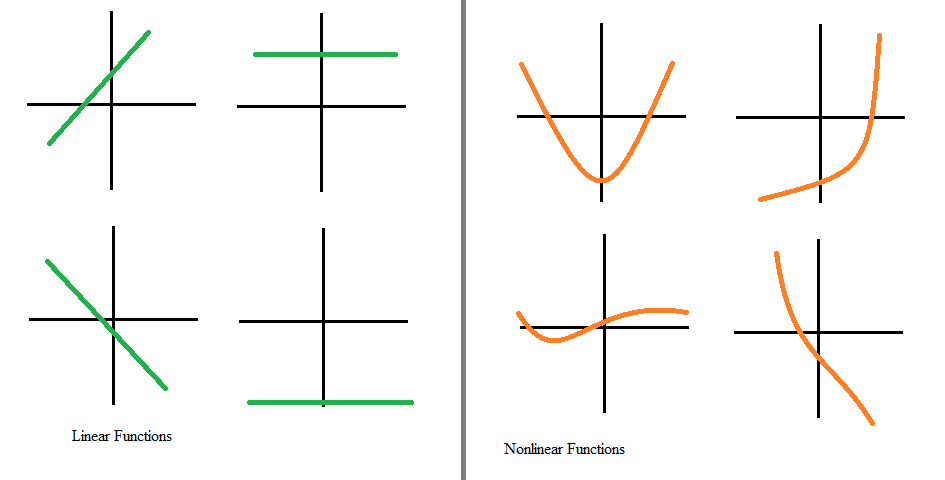Image 1: Linear Functions vs Non-linear functions from study.com

### Why is Activation function useful?

The activation function is biologically inspired by the activity in our brain where different neurons fire or activated by different stimuli. For eg. If we smell something pleasant like freshly baked cake certain neuron in our brain fire and become activated. If we smell something unpleasant like spoiled fruits this will cause other neurons in our brain to fire. So, within our brain certain neuron is either firing or not. This could be represented by ZERO (0) for not firing and ONE (1) for firing. A similar model is implemented in the activation function.  For example, in sigmoid 0 for not active and 1 for active. But in ReLU lower unit is 0 but the higher unit is the number itself. The idea is the more positive the neuron, the more activated it is. Activation functions for hidden layer and output layer could be different depending upon the type of the problem.Image 2: Sigmoid vs RELU activation function

### Why does your Neural Network (NN) need Non-Linear Activation Functions?

If we don’t use non-linear activation function no matter how many layers we use in the hidden layer, it will still behave just like a single layer. By using non-linear activation function the mapping of input to the output is non-linear. If you want to learn more please follow this link of Andrew Ng.

There are many activation functions among them the most popular are Sigmoid, tanH, Softmax, ReLU, Leaky ReLU.

### Identity or Linear

It is simple of all activation function because it outputs whatever the input is. ie. f(x) = xImage 3: Identity activation function

### Binary Step

If our input is greater than ZERO (0) than it gives you ONE (1) and if our input is less than ZERO (0) it gives ZERO(0). So, it takes all the positive input, and make it 1 and it takes all the negative input, and make it 0. It is very useful in classifiers when you want to classify between 0 and 1.

### Logistic or Sigmoid

Whatever the input this function maps between 0 to 1. So, even if your inputs are as large as hundred or thousands or millions, it maps between 0 and 1. Sigmoid takes in an input and if the input is very negative number than the sigmoid with transforming the input close to 0 and if the input is very positive number than the sigmoid will transform the input into a number very close to one 1. And, if the number is close to 0 than sigmoid will transform the input into some number between 0 and 1. So, for sigmoid 0 is the lower unit and 1 is the higher unit.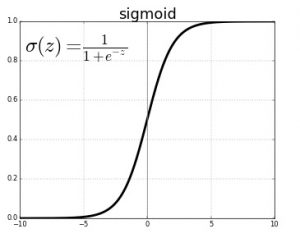If you are solving the problem of binary classification than sigmoid would be a good choice in the output layer. There are two drawbacks to the sigmoid activation function. The first one is vanishing gradient problem. When the input is highly positive or negative, the response saturates and the gradient is almost zero at these points. No gradients mean no converges, as a result, the model cannot find the ideal weights and training never converges to a good solution. Another one is output is not ZERO centered. It starts at 0 and ends at 1 that means the value after the function will be positive and that makes the gradient of the weight become either all positive or all negative. This makes the gradient updates go too far in a different direction which makes optimization harder. I have also attached the supporting solution from StackExchange by dontloo.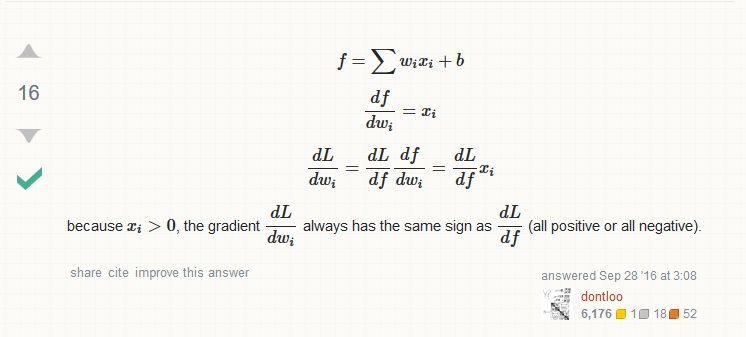### Tanh

Tanh is similar to sigmoid function the only difference is it squishes the real number into a range between -1 and 1 instead of 0 and 1. It looks like shifted and verticle scale form of a sigmoid function having the range between -1 and 1. Similar to sigmoid function vanishing gradient problem exists but its output is ZERO centered which makes optimization easier. Tanh works better than sigmoid function because, with values between -1 and +1, the mean of the activations that comes out of your hidden layer are closer to having a zero mean.ReLU (Rectified Linear Unit )

ReLU is most popular one when it comes to deep learning and even normal Neural Networks (NN). Whenever the function is less than zero it remains zero and whenever the function is greater than zero than it remains as it is.

f(x) = max(0,x)

Relu(x) = { 0 if x < 0; x if x >=0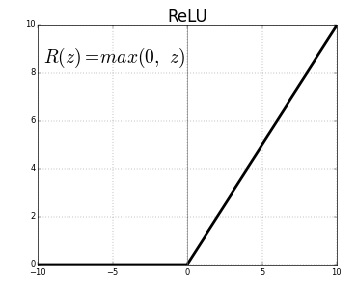Though it has two linear pieces. It is surprising when composed together through multiple successive hidden layers.

The main advantage is it removes the negative part of the function.

Leaky ReLU

This is similar to Leaky ReLU, the only difference is it does not make the negative input zero, it just reduces the magnitude of it.

Leaky ReLU (yi) = { 0 if x < 0; ax otherwise and a is typically very low (0.01)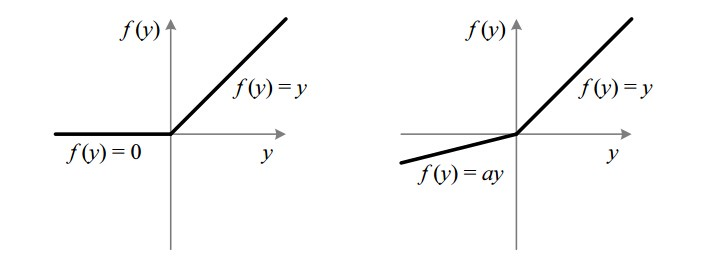### Softmax

The softmax function is a more generalized logistic activation function which is used for multiclass classification. This is used to impart probabilities. When you have four or five outputs and you pass through it you get the probability distribution of each. And this is useful for finding out most probable occurrence or the classification where the probability of a class is maximum. It is generally used in the last layer for classification problem. And, the right cost function to use with softmax is cross-entropy.

So these are some famous activation functions which are very useful and play an important role in deciding the accuracy of the model. Whenever you have doubted this activation functions might be better it’s always better that you use it and test the accuracy, because you never know which activation function will give you the best accuracy.